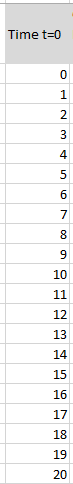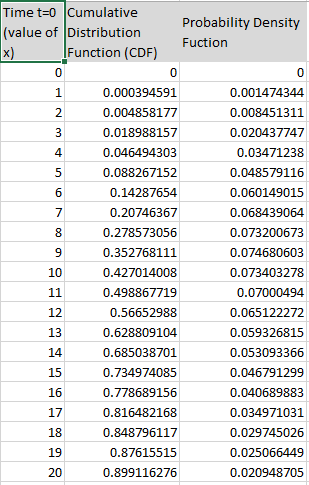## Gamma Distribution in Excel

In MS Excel, Gamma distribution can be easily calculated by using GAMMA.DIST function. This function is available from MS Excel 2010 onwards. For previous version there is GAMMADIST function (without a dot between).

Function Details

=GAMMA.DIST (x, alpha, beta, cumulative)

Arguments of Function

• X (Required)– The value at which gamma function would be evaluated
• Alpha / α (Required) – A parameter of the distribution used for determining the shape.
• beta / β (Required) – A parameter of the distribution for determining the rate

α and β are both must be greater than 1.

When α = 1, it corresponds to exponential distribution.

When β = 1, it corresponds to the standard gamma distribution.

Cumulative (Required) – A logical value that determines the form of the function.

Cumulative =TRUE, it returns the cumulative distribution function

Cumulative =FALSE, it returns the probability density function.

Stepwise Execution

Go to an empty cell and type =GAMMA.DIST1. Type the value where we need to find probability. This gives us the value of x. In the example below, x= 5
2. Type the alpha and beta value as the next parameters, both comma separated. Here, α = 4 & β = 3
3. Type True for cumulative distribution. Type False for probability density function.

Formula & Result

=GAMMA.DIST(5, 4, 3, TRUE)

Result: 0.088267

=GAMMA.DIST(5, 4, 3, False)

Result: 0.048579

Graph Generation Cumulative Distribution function (CDF) and Probability Density Function (PDF)

Take time T = 0 – n. In the example, time is taken from 0 to 20. Here value of T = value of xFix values for α = 4 & β = 3Cumulative Distribution function (CDF)

Formula: =GAMMA.DIST(D16, 4, 3, TRUE)

Probability Density function (PDF)

Formula: =GAMMA.DIST(D16, 4, 3, FALSE)Errors

• #NUM! – Occurs under following scenarios:

Value of x < 0

Value of α ≤ 0 or β ≤ 0

• #VALUE! – Occurs under following scenarios:

Any of the arguments – x, alpha, beta are non-numeric in nature

The fourth parameter is not TRUE OR FALSE, unrecognized

## Template

```Further reading: